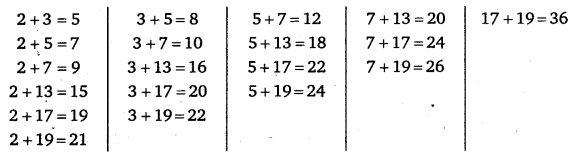# Class 6 Maths NCERT Solutions for Chapter 3 Playing with Numbers Ex 3.2

## Playing with Numbers

Question 1.
What is the sum of any two
(a)
Odd numbers?
(b) Even numbers?

Solution:
(a) Sum of two odd numbers is even.
(b) Sum of two even numbers is even.

Question 2.
State whether the following statements are True or False :
(a)
The sum of three odd numbers is even.
(b) The sum of two odd numbers and one even number is even.
(c) The product of three odd numbers is odd.
(d) If an even number is divided by 2, the quotient is always odd.
(e) All prime numbers are odd.
(f) Prime numbers do not have any factors.
(g) Sum of two prime numbers is always even.
(h) 2 is the only even prime number.
(i) All even numbers are composite numbers.
(j) The product of two even numbers is always even.

Solution:
(a) False
(b) True
(c) True
(d) False
(e) False
(f) False
(g) False
(h) True
(i) False
(j) True

Question 3.
The numbers 13 and 31 are prime numbers. Both these numbers have same digits 1 and 3. Find such pairs of prime numbers upto 100.

Solution:
By the Sieve of Eratosthenes method find the prime numbers between 1 and 100. We find that these are
2, 3, 5, 7, 11, 13, 17, 19, 23, 29, 31, 37, 41, 43, 47, 53, 59, 61, 67, 71, 73, 79, 83, 89 and 97.
Out of these, a pair of prime numbers having same digits are 13, 31; 17, 71; 37, 73, 79, 97.

Question 4.
Write down separately the prime and composite numbers less than 20.

Solution:
Prime numbers less than 20 are 2, 3, 5, 7, 11, 13, 17 and 19.
Composite numbers less than 20 are 4, 6, 8, 9, 10, 12, 14, 15, 16 and 18.

Question 5.
What is the greatest prime number between 1 and 10?

Solution:
Prime numbers between 1 and 10 are 2, 5 and 7.
∴ Greatest prime number between 1 and 10 = 7

Question 6.
Express the following as the sum of two odd primes
(a)
44
(b) 36
(c) 24
(d) 18

Solution:
(a) 44 = 13 + 31
(b) 36 = 5 + 31
(c) 24 = 11 +13
(d) 18 = 7+11
Note : In 1742, mathematician Goldbach had a conjecture (guess) for which he could not provide a proof. It may be stated as “Every even number greater than 4 can be expressed as the sum of two odd prime numbers”.

Question 7.
Give three pairs of prime numbers whose difference is 2.

Solution:
Three pairs of prime number whose difference is 2 are 3, 5; 5, 7 and 11, 13.
Note : Two prime numbers are known as twin-primes if there is one composite number between them. In other words, two prime numbers whose difference is 2 are called twin-primes.

Question 8.
Which of the following numbers are prime? ,
(a)
23
(b) 51
(c) 37
(d) 26

Solution:
(a) We find that 23 is not exactly divisible by any of the prime numbers 2, 3, 5, 7 and 11 (i.e., upto half of 23). So, it is a prime number.
(b) We find that 51 is divisible by 3. So, it is not a prime number.
(c) We find that 37 is not exactly divisible by any of the prime numbers 2, 3, 5, 7, 11, 13 and 17 (i.e., upto half of 37). So, it is a prime number.
(d) We find that 26 is exactly divisible by 2 and 13. So, it is not a prime number.

Question 9.
Write seven consecutive composite numbers less-than 100 so that there is no prime number between them.

Solution:
Seven consecutive composite numbers less than 100 so that there is no prime number between them are 90, 91, 92, 93, 94, 95 and 96.

Question 10.
Express each of the following numbers as the sum of three odd primes:
(a)
21
(b) 31
(c) 53
(d) 61

Solution:
Expressing the given numbers as the sum of three odd primes, we have
(a) 21 = 3 + 5 + 13
(b) 31 = 3 + 5 + 23
(c) 53 = 3 + 19 + 31
(d) 61 = 3 + 11 + 47

Question 11.
Write five pairs of prime numbers less than 20 whose sum is divisible by 5.

Solution:
Prime numbers below 20 are 2, 3, 5, 7, 13, 17 and 19.
Possible sum of pairs of these numbers :Clearly, five pairs of prime numbers whose sum is divisible by 5 are 2, 3; 2, 13; 3, 7; 3,17 and 7, 13.

Question 12.
Fill in the blanks :
(a)
A number which has only two factors is called a
(b) A number which has more than two factors is called a
(c) 1 is neither nor
(d) The smallest prime number is
(e) The smallest composite number is
(f) The smallest even number is

Solution:
(a) Prime
(b) composite
(c) prime, composite
(d) 2
(e) 4
(f) 2